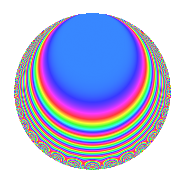# Properties

 Label 224.2.fLevel 224 Weight 2 Character orbit f Rep. character $$\chi_{224}(223,\cdot)$$ Character field $$\Q$$ Dimension 8 Newforms 1 Sturm bound 64 Trace bound 0

# Related objects

## Defining parameters

 Level: $$N$$ = $$224 = 2^{5} \cdot 7$$ Weight: $$k$$ = $$2$$ Character orbit: $$[\chi]$$ = 224.f (of order $$2$$ and degree $$1$$) Character conductor: $$\operatorname{cond}(\chi)$$ = $$28$$ Character field: $$\Q$$ Newforms: $$1$$ Sturm bound: $$64$$ Trace bound: $$0$$

## Dimensions

The following table gives the dimensions of various subspaces of $$M_{2}(224, [\chi])$$.

Total New Old
Modular forms 40 8 32
Cusp forms 24 8 16
Eisenstein series 16 0 16

## Trace form

 $$8q + 8q^{9} + O(q^{10})$$ $$8q + 8q^{9} + 8q^{25} - 16q^{29} - 16q^{37} + 8q^{49} - 16q^{53} - 32q^{57} - 32q^{65} - 16q^{77} - 24q^{81} + 64q^{85} + 64q^{93} + O(q^{100})$$

## Decomposition of $$S_{2}^{\mathrm{new}}(224, [\chi])$$ into irreducible Hecke orbits

Label Dim. $$A$$ Field CM Traces $q$-expansion
$$a_2$$ $$a_3$$ $$a_5$$ $$a_7$$
224.2.f.a $$8$$ $$1.789$$ $$\Q(\zeta_{16})$$ None $$0$$ $$0$$ $$0$$ $$0$$ $$q-\zeta_{16}^{2}q^{3}-\zeta_{16}^{5}q^{5}-\zeta_{16}^{6}q^{7}+\cdots$$

## Decomposition of $$S_{2}^{\mathrm{old}}(224, [\chi])$$ into lower level spaces

$$S_{2}^{\mathrm{old}}(224, [\chi]) \cong$$ $$S_{2}^{\mathrm{new}}(28, [\chi])$$$$^{\oplus 4}$$$$\oplus$$$$S_{2}^{\mathrm{new}}(112, [\chi])$$$$^{\oplus 2}$$